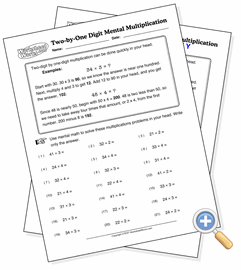# Two-by-One Digit Mental MultiplicationDevelop multiplication speed using estimation and differences

Some kinds of multiplication problems can be done faster in the head using estimation than on paper. Two-digit by one-digit multiplication is a prime example. By rounding up or down on the tens place to get a close value, the single-digit difference can be multiplied separately and either added or subtracted from the result. Use these problems to develop this extremely useful skill and end the slow pencil-and-paper multiplication.